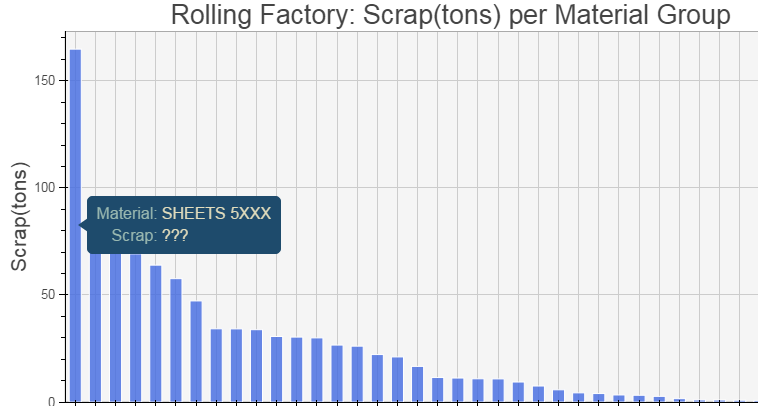# Hover Tool not working properly with bokeh.charts interface (Bar chart)

Hello,

I created a sorted bar chart using the bokeh.charts interface (bokeh 0.11) folowing this example: http://bokeh.pydata.org/en/latest/docs/gallery/stacked_bar_chart.html

However the Hover Tool does not seem to work properly:

import pandas as pd

from bokeh.charts import Bar

from bokeh.io import output_file, show

from bokeh.charts.attributes import cat

#Creating the DataFrame for chart 1: Filtering/Grouping/Sorting

df_chart1 = df_rolling[df_rolling.SCRAP>0]

df_chart1 = df_chart1.groupby(by=‘MATERIAL_GROUP’,

*as_index=False)[‘SCRAP’].sum() *

df_chart1 = df_chart1.sort_values(by=‘SCRAP’,ascending=False)

#Calculating the Scrap in tons

df_chart1[‘SCRAP_tn’] = df_chart1.SCRAP/1000

#Plotting chart 1

chart1 = Bar(df_chart1,values=‘SCRAP_tn’,

label=cat(columns=‘MATERIAL_GROUP’,sort=False),

title=‘Rolling Factory: Scrap(tons) per Material Group’,

ylabel=‘Scrap(tons)’,xlabel=‘Material Group’,

bar_width=0.6,xgrid=True,color=‘RoyalBlue’,

tooltips=[(‘Material’,’@MATERIAL_GROUP’),(‘Scrap’,’@SCRAP_tn’)])

chart1.title_text_font_size = ‘20pt’

chart1.background_fill_color = “whitesmoke”

chart1.plot_width=900

output_file(‘DMSY.html’)

show(chart1)

The result is the attached picture. I should be able to see the amount of scrap in tons (e.g. “165.4”) but all I see is “???”

Does anyone have any idea how to help me?